# 算法-字符串类问题

## 1. 重复的子字符串

  1 2 3 4 5 6 7 8 9 10 11 12 13 14 15  给定一个非空的字符串，判断它是否可以由它的一个子串重复多次构成。给定的字符串只含有小写英文字母，并且长度不超过10000。 示例 1: 输入: "abab" 输出: True 解释: 可由子字符串 "ab" 重复两次构成。 示例 2: 输入: "aba" 输出: False 示例 3: 输入: "abcabcabcabc" 输出: True 解释: 可由子字符串 "abc" 重复四次构成。 (或者子字符串 "abcabc" 重复两次构成。)

1. 字符串长度满足：0 < len(s) <= 10000，所以不需要考虑空字符串
2. 字符串的子串不包括自己，也就是说，「一个字符串由自己重复 1 次构成」这种说法不成立，可由示例2得出

1. 遍历所有可能的子串长度，从 1 到 len(s)/2，然后判断这个长度的子串是否可能为结果。取 len(s)/2 是因为超过字符串长度一半的子串不可能通过重复构成原字符串；
2. 字符串的长度一定是子串长度的倍数，否则也不可能是结果；
3. 考虑如何取给定长度的子串，由于字符串由子串重复构成，那么只需要从第一个字符开始取即可，长度为 n 的子串就从第一个字符开始取 n 个字符构成子串；

  1 2 3 4 5 6 7 8 9 10 11 12 13 14 15 16 17 18 19 20 21 22 23  func repeatedSubstringPattern(s string) bool { // 字符串长度可能是1到len(s)/2 for i := 1; i <= len(s)/2; i++ { // 只有 len(s) 是子字符串长度的倍数才有可能 if len(s) % i != 0 { continue } // 子串为 s[:i]，然后对字符串的其它部分进行判断 var flag bool for j := i; j < len(s); j += i { if s[j:j+i] != s[:i] { flag = true break } } // 中途没有跳出才说明整个字符串由子串重复构成 if !flag { return true } } // 所有长度的子串都无法重复构成原字符串 return false }

1. i <= len(s)/2：当 i == len(s)/2 的时候，子串为 s[:len(s)/2]，以字符串 abab 为例，子串为 ab，因为 Go 的切片不会取最后一个字符，所以必须添加 = 号，不然会漏掉一种情况；

2. j < len(s)：我们考虑最后一次循环的 j，此时 s[j:j+i]j+i 可能会越界超出 len(s)，导致 panic。遇到这种情况我们最常用的做法是修改条件为 j + i < len(s)，然后我们来考虑边界条件。

j + i == len(s) 的时候， s[j:j+i] 其实原本想表达的含义是最后一个字符串，是应该取的，但是 j + i 产生越界，所以要额外处理。

  1 2 3 4 5 6 7 8 9 10 11 12 13 14 15 16 17 18 19 20 21 22 23 24 25 26 27 28 29  func repeatedSubstringPattern(s string) bool { // 字符串长度可能是1到len(s)/2 for i := 1; i <= len(s)/2; i++ { // 只有 len(s) 是子字符串长度的倍数才有可能 if len(s) % i != 0 { continue } // 子串为 s[:i]，然后对字符串的其它部分进行判断 var flag bool for j := i; j + i <= len(s); j += i { var tmp string if j + i == len(s) { tmp = s[j:] }else{ tmp = s[j:j+i] } if tmp != s[:i] { flag = true break } } // 中途没有跳出才说明整个字符串由子串重复构成 if !flag { return true } } // 所有长度的子串都无法重复构成原字符串 return false }

## 2. 模式匹配BF算法

 1 2 3 4 5 6 7  示例 1: 输入: haystack = "hello", needle = "ll" 输出: 2 示例 2: 输入: haystack = "aaaaa", needle = "bba" 输出: -1

  1 2 3 4 5 6 7 8 9 10 11 12 13 14 15 16 17 18 19 20 21 22  func strStr(haystack string, needle string) int { // 子串为空，返回0 if len(needle) == 0 { return 0 } // = 号是考虑子串等于主串的情况 for i := 0; i + len(needle) <= len(haystack); i++ { var flag bool for j := 0; j < len(needle); j++ { if haystack[i+j] != needle[j] { flag = true break } } if !flag { return i } } return -1 }

## 3. 模式匹配KMP算法

S = abaabaabeca，T = abaabe，KMP 的流程如下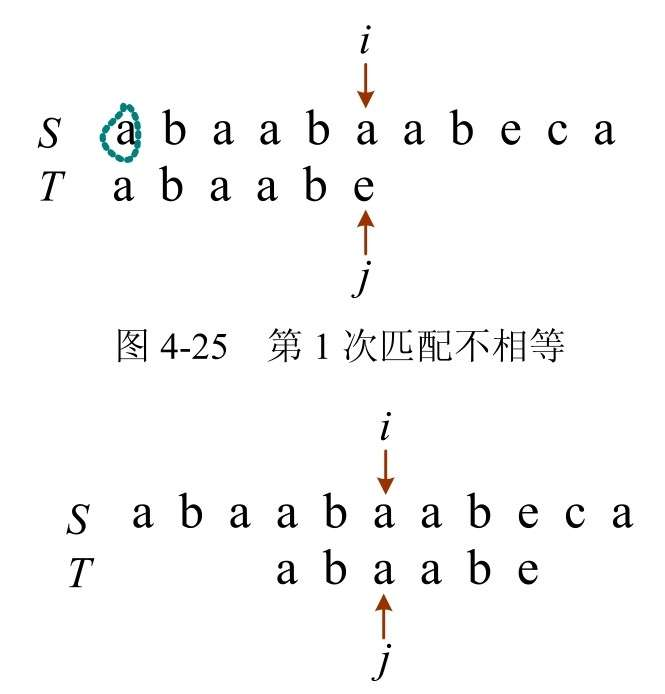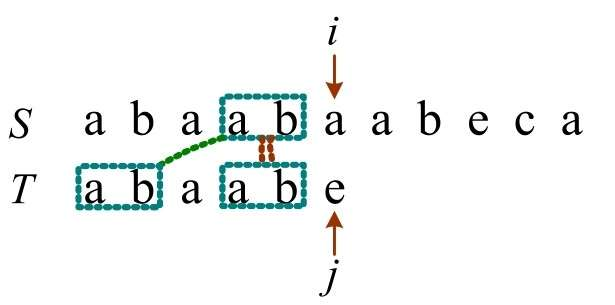1. 长度为1：前缀 a，后缀 b，不相等
2. 长度为2：前缀 ab，后缀 ab，相等
3. 长度为3：前缀 aba，后缀 aab，不相等
4. 长度为4：前缀 abaa，后缀 baab，不相等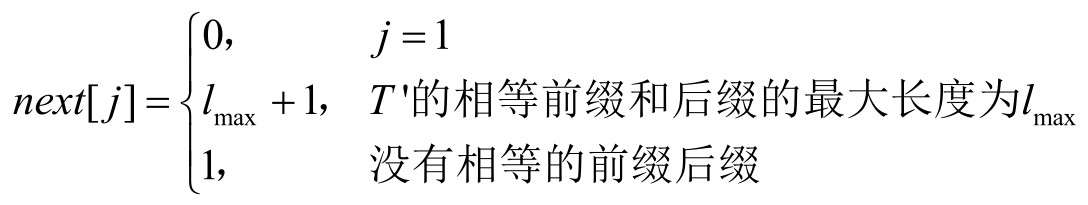j123456
Tabaabe
next[j]011223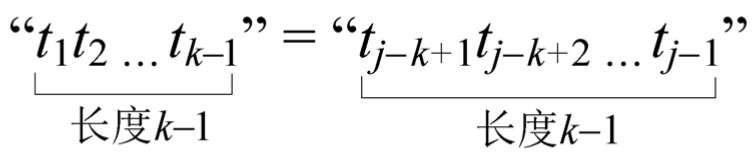1. $t_k = t_j$：那么 next[j+1] = k+1，即相等前缀和后缀的长度比 next[j] 多1，如下图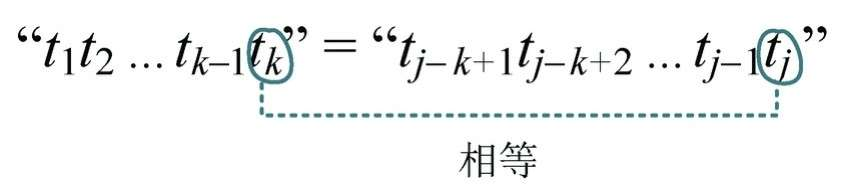2. $t_k \neq t_j$：那么回退找 next[k] = k’ 的位置，比较 $t_{k’}$ 和 $t_j$ 是否相等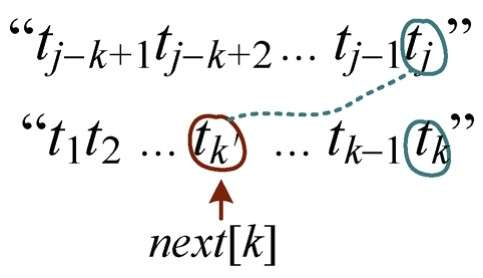如果相等，则 next[j+1] = k’’ + 1，如果不相等，继续向前找，直到找到 next[i] = 0 停止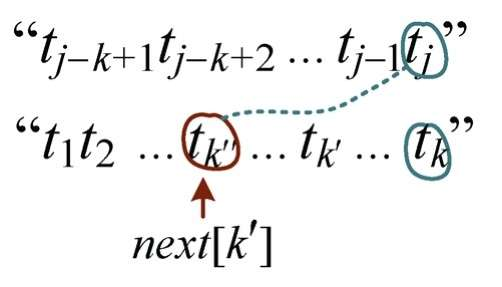1 2 3 4 5 6 7 8 9 10 11 12  func getNext(T string, next []int) { j,k := 1,0 next = 0 for j < len(T) { if k == 0 || T[j] == T[k] { j,k = j+1,k+1 next[j] = k }else{ k = next[k] } } }

KMP 算法的代码如下

  1 2 3 4 5 6 7 8 9 10 11 12 13 14 15 16  func KMP(S,T string, pos int, next []int) int { i,j := pos, 1 for i <= len(S) && j <= len(T) { if j == 0 || S[i] == T[j] { i++ j++ }else{ j = next[j] } } if j > len(T) { return i-len(T) }else{ return 0 } }支付宝微信